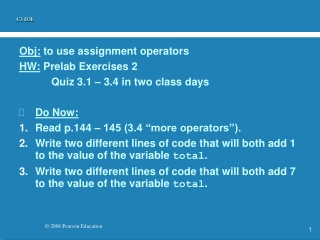DownloadDownload PresentationC3 D3b

# C3 D3b

Download Presentation## C3 D3b

- - - - - - - - - - - - - - - - - - - - - - - - - - - E N D - - - - - - - - - - - - - - - - - - - - - - - - - - -
##### Presentation Transcript

1. C3 D3b Obj: to use assignment operators HW: Prelab Exercises 2 Quiz 3.1 – 3.4 in two class days • Do Now: • Read p.144 – 145 (3.4 “more operators”). • Write two different lines of code that will both add 1 to the value of the variable total. • Write two different lines of code that will both add 7 to the value of the variable total.

2. Increment and Decrement • The increment and decrement operators are arithmetic and operate on one operand • The increment operator (++) adds one to its operand • The decrement operator (--) subtracts one from its operand • The statement count++; is functionally equivalent to count = count + 1;

3. Assignment Operators • Often we perform an operation on a variable, and then store the result back into that variable • Java provides assignment operators to simplify that process • For example, the statement num += count; is equivalent to num = num + count;

4. Operator += -= *= /= %= Example x += y x -= y x *= y x /= y x %= y Equivalent To x = x + y x = x - y x = x * y x = x / y x = x % y Assignment Operators • There are many assignment operators, including the following:

5. Assignment Operators • The entire right-hand side of an assignment expression is evaluated first, then the result is combined with the original variable • Therefore result /= (total-MIN) % num; is equivalent to result = result / ((total-MIN) % num);

6. Try this: • p.180 Multiple Choice #3.1 • If time: begin “Processing Grades” Lab.